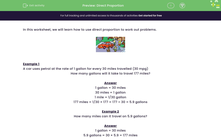# Use Direct Proportion to Solve Problems

In this worksheet, students will study direct proportion.Key stage:  KS 3

Curriculum topic:   Ratio, Proportion and Rates of Change

Curriculum subtopic:   Solve Problems Involving Direct and Inverse Proportion

Popular topics:   Ratio worksheets

Difficulty level:#### Worksheet Overview

In this activity, we will learn how to use direct proportion to work out problems.Example 1

A car uses petrol at the rate of 1 gallon for every 30 miles travelled (30 mpg)

How many gallons will it take to travel 177 miles?

1 gallon = 30 miles
30 miles = 1 gallon
1 mile = 1/30 gallon
177 miles = 1/30 × 177 = 177 ÷ 30 = 5.9 gallons

Example 2

How many miles can it travel on 5.9 gallons?

1 gallon = 30 miles
5.9 gallons = 30 × 5.9 = 177 miles

Does that make sense? Let's try some questions together now.

### What is EdPlace?

We're your National Curriculum aligned online education content provider helping each child succeed in English, maths and science from year 1 to GCSE. With an EdPlace account you’ll be able to track and measure progress, helping each child achieve their best. We build confidence and attainment by personalising each child’s learning at a level that suits them.

Get started